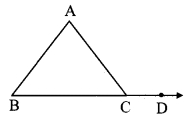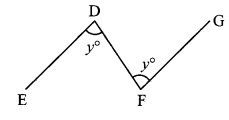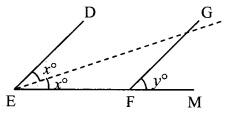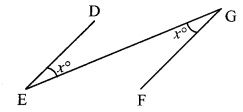# Maharashtra Board 9th Class Maths Part 2 Practice Set 3.1 Solutions Chapter 3 Triangles

Balbharti Maharashtra State Board Class 9 Maths Solutions covers the Practice Set 3.1 Geometry 9th Class Maths Part 2 Answers Solutions Chapter 3 Triangles.

## Practice Set 3.1 Geometry 9th Std Maths Part 2 Answers Chapter 3 Triangles

Practice Set 3.1 Geometry 9th Standard Question 1.
In the adjoining figure, ∠ACD is an exterior angle of ∆ABC. ∠B = 40°, ∠A = 70°. Find the measure of ∠ACD.Solution:
∠A = 70° , ∠B = 40° [Given]
∠ACD is an exterior angle of ∆ABC. [Given]
∴ ∠ACD = ∠A + ∠B
= 70° + 40°
∴ ∠ACD = 110°

Question 2.
In ∆PQR, ∠P = 70°, ∠Q = 65°, then find ∠R.
Solution:
∠P = 70°, ∠Q = 65° [Given]
In ∆PQR,
∠P + ∠Q + ∠R = 180° [Sum of the measures of the angles of a triangle is 180°]
∴ 70° + 65° + ∠R = 180°
∴ ∠R = 180° – 70° – 65°
∴ ∠R = 45°

Practice Set 3.1 Geometry 9th Question 3.
The measures of angles of a triangle are x°, (x – 20)°, (x – 40)°. Find the measure of each angle.
Solution:
The measures of the angles of a triangle are x°, (x – 20)°, (x – 40)°. [Given]
∴ x°+ (x – 20)° + (x – 40)° = 180° [Sum of the measures of the angles of a triangle is 180°]
∴ 3x – 60 = 180
∴ 3x = 180 + 60
∴ 3x = 240
∴ x = 240
∴ x = $$\frac { 240 }{ 3 }$$
∴ x = 80°
∴ The measures of the remaining angles are
x – 20° = 80° – 20° = 60°,
x – 40° = 80° – 40° = 40°
∴ The measures of the angles of the triangle are 80°, 60° and 40°.

9th Class Geometry Practice Set 3.1 Question 4.
The measure of one of the angles of a triangle is twice the measure of its smallest angle and the measure of the other is thrice the measure of the smallest angle. Find the measures of the three angles.
Solution:
Let the measure of the smallest angle be x°.
One of the angles is twice the measure of the smallest angle.
∴ Measure of that angle = 2x°
Another angle is thrice the measure of the smallest angle.
∴ Measure of that angle = 3x°
∴ The measures of the remaining two angles are 2x° and 3x°.
Now, x° + 2x° + 3x° = 180° [Sum of the measures of the angles of a triangle is 180°]
∴ 6x = 180
∴ x = 180
∴ x = $$\frac { 180 }{ 6 }$$
∴ x° = 30°
The measures of the remaining angles are 2x° = 2 x 30° = 60°
3x° = 3 x 30° = 90°
The measures of the three angles of the triangle are 30°, 60° and 90°.

Question 5.
In the adjoining figure, measures of some angles are given. Using the measures, find the values of x, y, z.Solution:
i. ∠NET = 100° and ∠EMR = 140°
∠EMN + ∠EMR = 180°
∴ z +140° =180°
∴ z = 180° -140°
∴ z = 40°

ii. Also, ∠NET + ∠NEM = 180° [Angles in a linear pair]
∴ 100° + y = 180°
∴ y = 180° – 100°
∴ y = 80°

iii. In ∆ENM,
∴ ∠ENM + ∠NEM + ∠EMN = 180° [Sum of the measures of the angles of a triangle is 180°]
∴ x +80°+ 40°= 180°
∴ x = 180° – 80° – 40°
∴ x = 60°
∴ x = 60°, = 80°, z = 40°

Question 6.
In the adjoining figure, line AB || line DE. Find the measures of ∠DRE and ∠ARE using given measures of some angles.Solution:
i. ∠B AD = 70°, ∠DER = 40° [Given]
line AB || line DE and seg AD is their transversal.
∴ ∠EDA = ∠BAD [Alternate Angles]
∴ ∠EDA = 70° ….(i)
In ∆DRE,
∠EDR + ∠DER + ∠DRE = 180° [Sum of the measures of the angles of a triangle is 180°]
∴ 70°+ 40° +∠DRE = 180° [From (i) and D – R – A]
∴ ∠DRE = 180° -70° -40°
∴ ∠DRE = 70°

ii. ∠DRE + ∠ARE = 180° [Angles in a linear pair]
∴ 70° + ∠ARE = 180°
∴ ∠ARE = 180°-70°
∴ ∠ARE =110°
∴ ∠DRE = 70°, ∠ARE = 110°

Triangles Class 9 Practice Set 3.1 Question 7.
In ∆ABC, bisectors of ∠A and ∠B intersect at point O. If ∠C = 70°, find the measure of ∠AOB.
Solution:
∠OAB = ∠OAC = – ∠BAC ….(i) [Seg AO bisects ∠BAC]
∠OBA = ∠OBC = – ∠ABC …..(ii) [Seg RO bisects ∠ABC]
In AABC,
∠BAC + ∠ABC + ∠ACB = 180° [Sum of the measures of the angles of a triangle is 180°]∴ ∠BAC + ∠ABC + 70° = 180°
∴ ∠BAC + ∠ABC = 180°- 70°
∴ ∠BAC + ∠ABC = 110°
∴ $$\frac { 1 }{ 2 }$$(∠BAC) + $$\frac { 1 }{ 2 }$$ (∠ABC) = $$\frac { 1 }{ 2 }$$ x 110° [MuItiplying both sides by $$\frac { 1 }{ 2 }$$]
∴ ∠OAB + ∠OBA = 55° ….(iii) [From (i) and (ii)]
In AOAB,
∠OAB + ∠OBA + ∠AOB = 180° [Sum of the measures of the angles of a triangle is 180°]
∴ 55° + ∠AOB = 180° [From (iii)]
∴ ∠AOB = 180°- 55°
∴ ∠AOB = 125°

Question 8.
In the adjoining figure, line AB || line CD and line PQ is the transversal. Ray PT and ray QT are bisectors of ∠BPQ and ∠PQD respectively. Prove that m ∠PTQ = 90°.Given: line AB || line CD and line PQ is the transversal.
ray PT and ray QT are the bisectors of ∠BPQ and ∠PQD respectively.
To prove: m∠PTQ = 90°
Solution:
Proof:
∠TPB = ∠TPQ = $$\frac { 1 }{ 2 }$$∠BPQ …(i) [Ray PT bisects ∠BPQ]
∠TQD = ∠TQP = $$\frac { 1 }{ 2 }$$∠PQD ….(ii) [Ray QT bisects ∠PQD]
line AB || line CD and line PQ is their transversal. [Given]
∴∠BPQ + ∠PQD = 180° [Interior angles]
∴ $$\frac { 1 }{ 2 }$$ (∠BPQ) + $$\frac { 1 }{ 2 }$$ (∠PQD) = $$\frac { 1 }{ 2 }$$ x 180° [Multiplying both sides by $$\frac { 1 }{ 2 }$$]
∠TPQ + ∠TQP = 90°
In ∆PTQ,
∠TPQ + ∠TQP + ∠PTQ = 180° [Sum of the measures of the angles of a triangle is 180°]
∴ 90° + ∠PTQ = 180° [From (iii)]
∴ ∠PTQ = 180° – 90°
= 90°
∴ m∠PTQ = 90°

Triangle Practice Set 3.1 Question 9.
Using the information in the adjoining figure, find the measures of ∠a, ∠b and ∠c.Solution:
i. ∠c + 100° = 180° [Angles in a linear pair]
∴ ∠c = 180° – 100°
∴ ∠c = 80°

ii. ∠b = 70° [Vertically opposite angles]
iii. ∠a + ∠b +∠c = 180° [Sum of the measures of the angles of a triangle is 180°]
∠a + 70° + 80° = 1800
∴ ∠a = 180° – 70° – 80°
∴ ∠a = 30°
∴ ∠a = 30°, ∠b = 70°,∠ c = 80°

Practice Set 3.1 Geometry Question 10.
In the adjoining figure, line DE || line GF, ray EG and ray FG are bisectors of ∠DEF and ∠DFM respectively. Prove that,i. ∠DEG = $$\frac { 1 }{ 2 }$$ ∠EDF
ii. EF = FG
Solution:
i. ∠DEG = ∠FEG = x° ….(i) [Ray EG bisects ∠DEF]
∠GFD = ∠GFM = y° …..(ii) [Ray FG bisects ∠DFM]
line DE || line GF and DF is their transversal. [Given]∴ ∠EDF = ∠GFD [Alternate angles]
∴ ∠EDF = y° ….(iii) [From (ii)]
line DE || line GF and EM is their transversal. [Given]∴ ∠DEF = ∠GFM [Corresponding angles]
∴ ∠DEG + ∠FEG = ∠GFM [Angle addition property]
∴ x°+ x° = y° [From (i) and (ii)]
∴ 2x° = y°
∴ x° = $$\frac { 1 }{ 2 }$$y°
∴ ∠DEG = $$\frac { 1 }{ 2 }$$∠EDF [From (i) and (iii)]

ii. line DE || line GF and GE is their transversal. [Given]∴ ∠DEG = ∠FGE …(iv) [Alternate angles]
∴ ∠FEG = ∠FGE ….(v) [From (i) and (iv)]
∴ In ∆FEG,
∠FEG = ∠FGE [From (v)]
∴ EF = FG [Converse of isosceles triangle theorem]

Maharashtra Board Class 9 Maths Chapter 3 Triangles Practice Set 3.1 Intext Questions and Activities

Class 9 Geometry Practice Set 3.1 Question 1. Can you give an alternative proof of the above theorem by drawing a line through point R and parallel to seg PQ in the above figure? (Textbook pg. no. 25)Solution:
Yes.
Construction: Draw line RM parallel to seg PQ through a point R.
Proof:
seg PQ || line RM and seg PR is their transversal. [Construction]
∴ ∠PRM = ∠QPR ……..(i) [Alternate angles]
seg PQ || line RM and seg QR is their transversal. [Construction]
∴ ∠SRM = ∠PQR ……..(ii) [Corresponding angles]
∴ ∠PRM + ∠SRM = ∠QPR + ∠PQR [Adding (i) and (ii)]
∴ ∠PRS = ∠PQR + ∠QPR [Angle addition property]

3 Triangles Question 2. Observe the given figure and find the measures of ∠PRS and ∠RTS. (Textbook pg. no.25)Solution:
∠PRS is an exterior angle of ∆PQR.
So from the theorem of remote interior angles,
∠PRS = ∠PQR + ∠QPR
= 40° + 30°
∴ ∠PRS = 70°
∴ ∠TRS=70° …[P – T – R]
In ∆RTS,
∠TRS + ∠RTS + ∠TSR = 180° …[Sum of the measures of the angles of a triangle is 180°]
∴ 70° + ∠RTS + 20° = 180°
∴ ∠RTS + 90° = 180°
∴ ∠RTS = 180°
∴ ∠RTS = 90°

9th Class Geometry Triangles Question 3. In the given figure, bisectors of ∠B and ∠C of ∆ABC intersect at point P. Prove that ∠BPC = 90° + $$\frac { 1 }{ 2 }$$∠BAC.
Complete the proof by filling in the blanks. (Textbook pg. no.27)Solution:
Proof:
In ∆ABC,
∠BAC + ∠ABC + ∠ACB = 180° …[Sum of the measures of the angles of a triangle is 180°]
∴ ∠BAC + – ∠ABC + ∠ACB = 180 … [Multiplying each term by $$\frac { 1 }{ 2 }$$]
∴ ∠BAC + ∠PBC + ∠PCB = 90°
∴ ∠PBC + ∠PCB = 90° – 1 ∠BAC ………(i)
In∆BPC,
∠BPC + ∠PBC + ∠PCB = 180° …….[Sum of measures of angles of a triangle]
∴ ∠BPC + 90° – $$\frac { 1 }{ 2 }$$∠BAC = 180° ……[From (i)]
∴ ∠BPC = 180° – 90°$$\frac { 1 }{ 2 }$$∠BAC
= 180°- 90°+ $$\frac { 1 }{ 2 }$$∠BAC
= 90°+ $$\frac { 1 }{ 2 }$$∠BAC